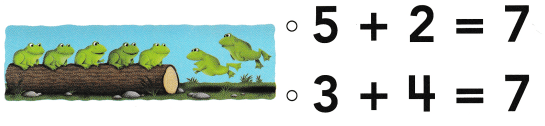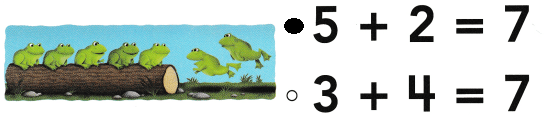# Texas Go Math Kindergarten Lesson 13.6 Answer Key Addition Word Problems

Refer to our Texas Go Math Kindergarten Answer Key Pdf to score good marks in the exams. Test yourself by practicing the problems from Texas Go Math Kindergarten Lesson 13.6 Answer Key Addition Word Problems.

## Texas Go Math Kindergarten Lesson 13.6 Answer Key Addition Word Problems

Essential Question
How can you solve addition word problems and complete the addition sentence?

Explore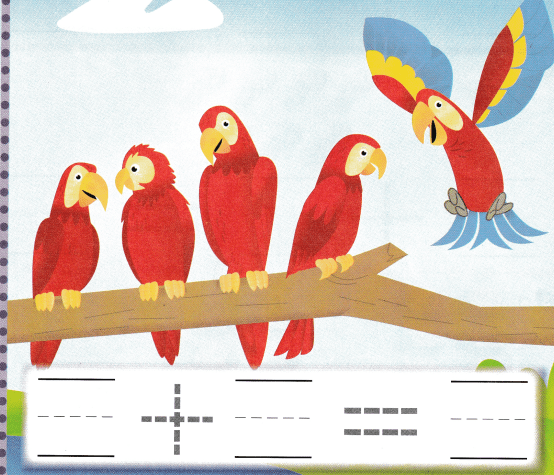Explanation:
4 birds sitting on a tree
1 more bird joined them
4 + 1 = 5
so, there are 5 birds in all.

Directions
Listen to the addition word problem about the birds. How many birds are on the tree? Write the number. How many birds join them? Write the number. Write the number of birds in all.

Try Another Problem

Question 1.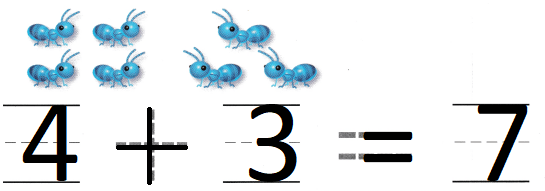Explanation:
There are 4 ants
3 more ants joined them
so, 7 ants in all
4 + 3 = 7
the sum of 4 and 3 is 7

Question 2.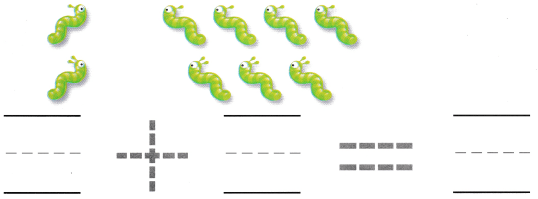Explanation:
There are 2 worms
and 7 more worms joined them
2 + 7 = 9
so, 9 worms in all.

Question 3.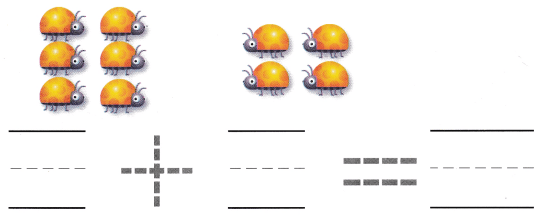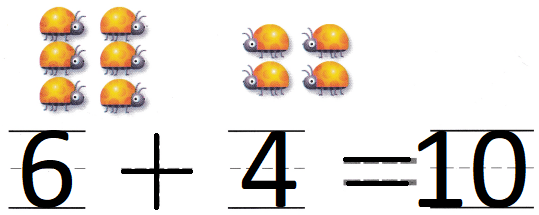Explanation:
and 4 more joined them
6 + 4 = 10
so, 10 bugs in all.

Directions
1-3. Listen to the addition word problem. How many are in the set to start with? Write the number. Write to complete the addition sentence.

Share and Show

Question 4.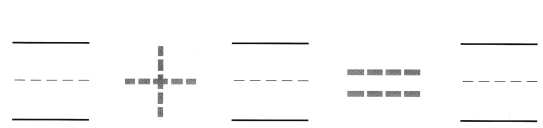Explanation:
There are 6 cars
and 3 more cars joined them
6 + 3 = 9
so, there are 9 cars in all.

Question 5.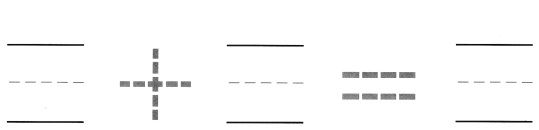Explanation:
There are 5 balls and
3 more balls joined them
5 + 3 = 8
so, 8 balls in all.

Question 6.Explanation:
There are 5 cherries
and 5 more in the box
5 + 5 = 10
so, 10 cherries in all.

Directions
4-6. Listen to the addition word problem. Draw to show how many are in the set to start with, Write the number, Draw to show how many are being added. Write the number. Write the number in all.

Question 7.Explanation:
There are five caterpillars.
Two more join them.
5 + 2 = 7
7  caterpillars are there now

Question 8.Explanation:
Tom puts 5 toy bugs in the box.
There were 4 bugs already in the box.
5 + 4 = 9
9 bugs are there now

Question 9.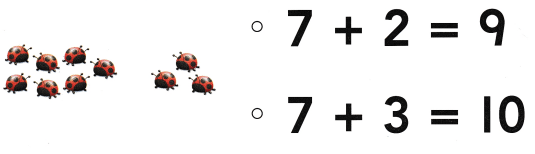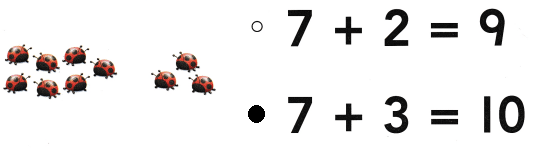Explanation:
Seven ladybugs are in a group.
3 more joined them
7 + 3 = 10
so, 10 bugs in all.

Directions
Choose the correct answer. 7. There are five caterpillars. Two more join them. How many caterpillars are there now? 8. Tom puts 5 toy bugs in the box. There were 4 bugs already in the box. How many bugs are there now? 9. Seven ladybugs are in a group. More ladybugs join them. What number sentence does the picture show?

### Texas Go Math Kindergarten Lesson 13.6 Homework and Practice

Question 1.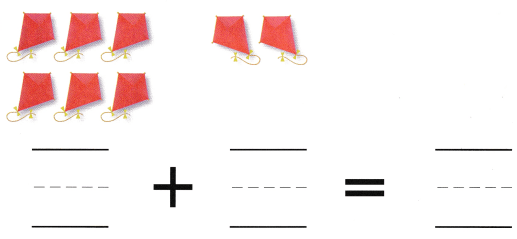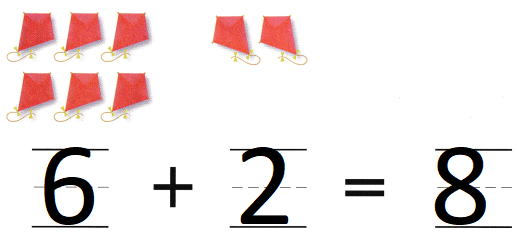Explanation:
There are six kites flying in the sky.
Then two more kites come flying
6 + 2 = 8
so, 8 kites are flying in the sky.

Question 2.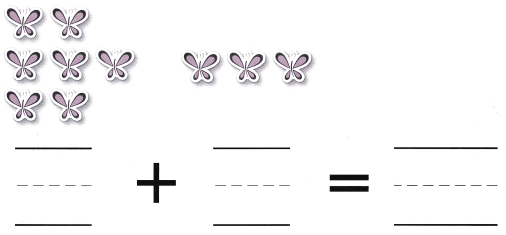Explanation:
There are seven butterflies.
Then three more join them.
7 + 3 = 10
so, 10 butter flies in all.

Directions
Listen to the addition word problem. How many are in the set to start with? Write the number. Write to complete the addition sentence. 1. There are six kites flying in the sky. Then two more kites come flying by. 2. There are seven butterflies. Then three more join them.

Texas Test Prep

Lesson Check

Question 3.Explanation:
. Bill’s mom buys 4 red apples.
4 + 2 = 6

Question 4.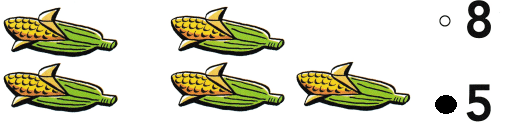Explanation:
Alan picks 2 ears of corn.
Then he picks 3 more ears of corn.
2 + 3 = 5
5 ears of corn that Alan pick

Question 5.